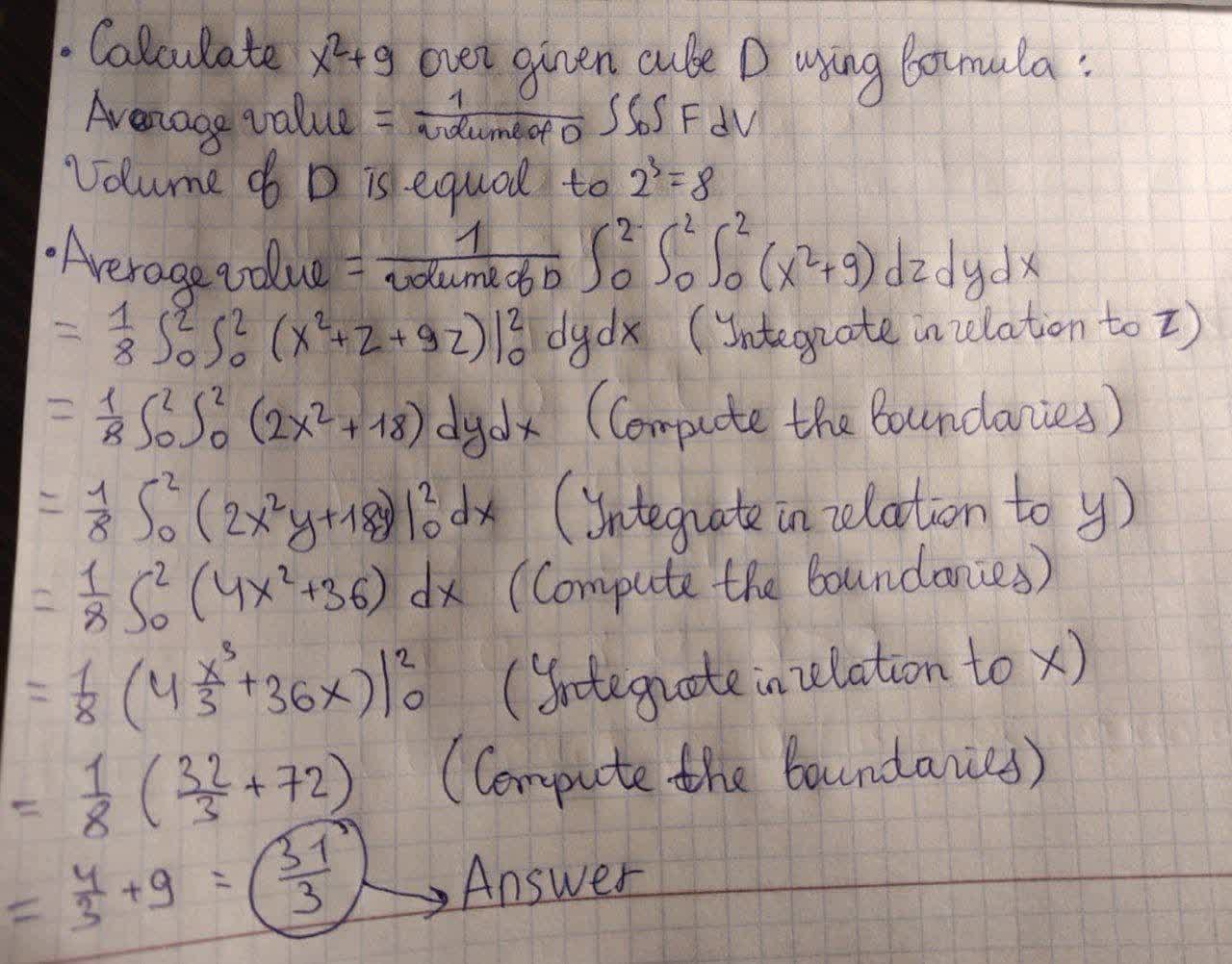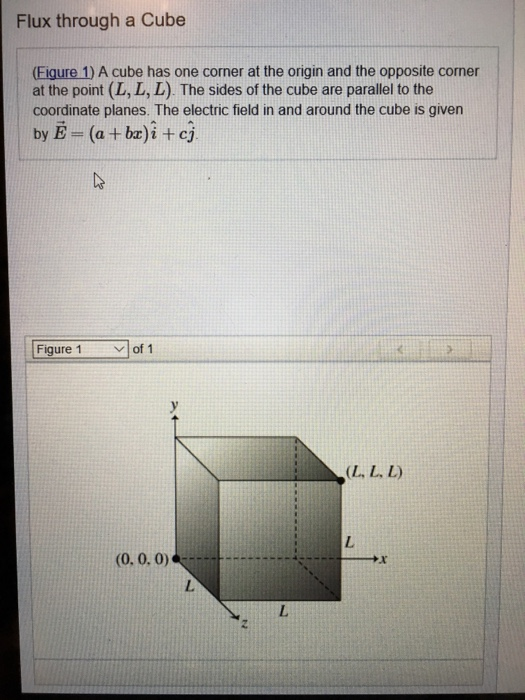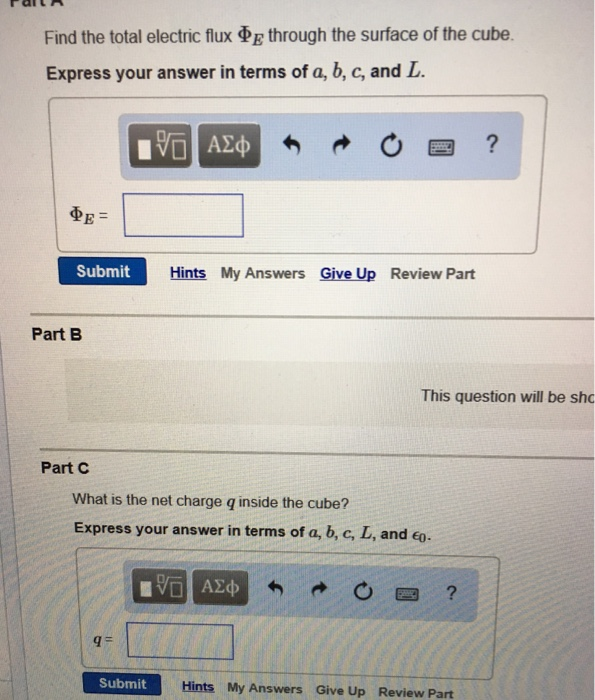Ask question

# Find the average value of F(x, y, z) over the given region. F(x, y, z) = x2 + 9 over the cube in the first octant bounded by the coordinate planes and the planes x = 2, y = 2, and z =2.# Find the average value of F(x, y, z) over the given region. F(x, y, z) = x2 + 9 over the cube in the first octant bounded by the coordinate planes and the planes x = 2, y = 2, and z =2.

Question
Negative numbers and coordinate planeasked 2021-02-24
Find the average value of F(x, y, z) over the given region. F(x, y, z) = x2 + 9 over the cube in the first octant bounded by the coordinate planes and the planes x = 2, y = 2, and z =2.

## Answers (1)2021-02-25
Let D be the volume of the cube bounded by the coordinate planes and the planes x = 2, y = 2, and z =2.
Then D = 2 * 2 * 2 = 8
Calculate average value of $$\displaystyle{x}^{{2}}+{9}$$ over give cube D using formula:
Average value $$\displaystyle=\frac{{1}}{{{v}{o}{l}{u}{m}{e}\ {o}{f}\ {D}}}\int\int_{{D}}\int{F}{d}{V}$$
Volume of D is equal to $$\displaystyle{2}^{{3}}={8}$$
Average value $$\displaystyle=\frac{{1}}{{{v}{o}{l}{u}{m}{e}\ {o}{f}\ {D}}}{\int_{{0}}^{{2}}}{\int_{{0}}^{{2}}}{\int_{{0}}^{{2}}}{\left({x}^{{2}}+{9}\right)}{\left.{d}{z}\right.}{\left.{d}{y}\right.}{\left.{d}{x}\right.}$$
$$\displaystyle=\frac{{1}}{{8}}{\int_{{0}}^{{2}}}{\int_{{0}}^{{2}}}{\left({x}^{{2}}{z}+{9}{z}\right)}{{\mid}_{{0}}^{{2}}}{\left.{d}{y}\right.}{\left.{d}{x}\right.}$$ (Integrate in relation to z.)
$$\displaystyle=\frac{{1}}{{8}}{\int_{{0}}^{{2}}}{\int_{{0}}^{{2}}}{\left({2}{x}^{{2}}+{18}\right)}{\left.{d}{y}\right.}{x}{e}$$ (Compute the boundaries)
$$\displaystyle=\frac{{1}}{{8}}{\int_{{0}}^{{2}}}{\left({2}{x}^{{2}}{y}+{18}{y}\right)}{{\mid}_{{0}}^{{2}}}{\left.{d}{x}\right.}$$ (Intergrate in relation to y)
$$\displaystyle=\frac{{1}}{{8}}{\int_{{0}}^{{2}}}{\left({4}{x}^{{2}}+{36}\right)}{\left.{d}{x}\right.}$$ (Compute the boundaries)
$$\displaystyle=\frac{{1}}{{8}}{\left({4}\frac{{x}^{{3}}}{{3}}+{36}{x}\right)}{{\mid}_{{0}}^{{2}}}$$ (Intergrate in relation to x)
$$\displaystyle=\frac{{1}}{{8}}{\left(\frac{{32}}{{3}}+{72}\right)}$$ (Compute the boundaries)
$$\displaystyle=\frac{{4}}{{3}}+{9}=\frac{{31}}{{3}}$$
Final answer $$\displaystyle=\frac{{31}}{{3}}$$### Relevant Questionsasked 2021-02-24
Write inequalities to describe the sets The solid cube in the first octant bounded by the coordinate planes and the planes x = 2, y = 2, and z = 2asked 2021-01-13
Average value over a multivariable function using triple integrals. Find the average value of $$\displaystyle{F}{\left({x},{y},{z}\right)}={x}^{{2}}+{y}^{{2}}+{z}^{{2}}$$ over the cube in the first octant bounded bt the coordinate planes and the planes x=5, y=5, and z=5asked 2021-02-25
Determine the volume of the largest box in the first octant with three in the coordinate planes, one vertex at the origin, and its opposite vertex in the plane x+3y+5z=15asked 2020-11-27
Obtain the volume of the solid which is bounded by a circular paraboloid $$\displaystyle{z}={x}^{{2}}+{y}^{{2}}$$, cylinder $$\displaystyle{x}^{{2}}+{y}^{{2}}={4}$$, and Coordinate plane. And the solid is in the $$\displaystyle{\left({x}\ge{0},{y}\ge{0},{z}\ge{0}\right)}$$.asked 2021-04-25
Flux through a Cube (Eigure 1) A cube has one corner at the origin and the opposite corner at the point (L, L, L). The sides of the cube are parallel to the coordinate planesasked 2020-10-27
Find the points of intersection of the line x = 3+2t, y =5+7t, z = -3+t, that is, I(t) = 3+2t, 5+7t, -3+t, with the coordinate planes.asked 2021-01-28
The volume of the largest rectangular box which lies in the first octant with three faces in the coordinate planes and its one of the vertex in the plane $$\displaystyle{x}+{2}{y}+{3}{z}={6}$$ by using Lagrange multipliers.asked 2021-01-31
Flux integrals Compute the outward flux of the following vector fields across the given surfaces S. You should decide which integral of the Divergence Theorem to use.
$$\displaystyle{F}={\left\langle{x}{\sin{{y}}},-{\cos{{y}}},{z}{\sin{{y}}}\right\rangle}$$ , S is the boundary of the region bounded by the planes x = 1, y = 0, $$\displaystyle{y}=\frac{\pi}{{2}},{z}={0}$$, and z = x.asked 2020-11-30
A cube is located with one corner at the origin of anx, y, z, coordinate system. One of the cube'sfaces lies in the x, y plane, another in they, z plane, and another in the x, zplane. In other words, the cube is in the first octant of thecoordinate system. The edges of the cube are 0.18 m long. A uniform electric field is parallelto the x, y plane and points in the direction of the+y axis. The magnitude of the field is 1500 N/C. Find the electric flux through each ofthe six faces of the cube. bottom face
Nm2/C
top face
Nm2/C
each of the other four faces
Nm2/Casked 2021-02-20
Set up the integral for the divergence theorem both ways. Then find the flux.
$$\displaystyle{F}{\left({x},{y},{z}\right)}={3}{x}\hat{{{i}}}+{x}{y}\hat{{{j}}}+{2}{x}{z}\hat{{{k}}}$$
E is the cube bounded by the planes x = 0, x = 3, y = 0, y = 3, and z = 0, z = 3.
...